# PySAL is still the fastest topology constructer in the west¶

One incredibly common question in spatial data analysis is the computation of adjacency relationships. Specifically, given a set of polygons, it's often common to ask queries like:

What polygons touch this polygon?



Or, in language you might actually know:

What neighborhoods surround mine?



These questions are usually specified as spatial predicates, and are computed in specialized database software. When answering these questions for statistical analysis, it's often important to have the answer to these queries over all pairs of observations. Thus, for geographic data science, we often require topology representing the spatial relationships between our observations. In polygonal data, the graph expressing the topological relationships between the polygons themselves is sometimes called the line graph or dual graph of the polygonal lattice.

Take, for instance, the common case of feature engineering in geographic data science. Here, we aim to use information about the geography of our data to get "free" data. Using the spatial embedding, we come up with additional information that might be helpful in predicting our outcomes of interest. So, for some data matrix $\mathbf{X}$, we'd like some function $\eta(\mathbf{x}_i)$, that gives a summary of all our features in nearby locations to site $i$. We could compute these averages by repeating many database queries. It's easier, though, to use some weights matrix, $\mathbf{W}$, whose product $\mathbf{WX}$ gives a summary of the values around each site. If you're interested in more, check out our tutorial on geographic spatial data science.

PySAL, the python spatial analysis library, has long provided fast methods for computing adjacency relationships from geometric data. This is important, since the computation of pairs of spatial relationships between neighboring pairs of observations can be quite time consuming if special structures are not recognized and exploited.

So, below, I'll show what these weights look like, and provide an example of how PySAL can be nearly an order of magnitude faster than using default geopandas, even with a spatial index.

In :
# this only works on the recent PySAL 2.0 release candidate!
from pysal.lib import weights, examples
import geopandas
import matplotlib.pyplot as plt
import numpy as np
%matplotlib inline


To do this, let's consider a dataset which is (in some senses) quite small: the set of all counties in the US:

In :
counties = geopandas.read_file(examples.get_path('NAT.shp'))
texas = counties.query("STATE_NAME == 'Texas'")

In :
counties.plot()

Out:
<matplotlib.axes._subplots.AxesSubplot at 0x7f064d798cc0>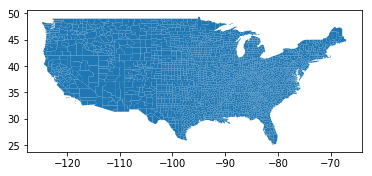While this is only:

In :
counties.shape

Out:
3085

observations, we can find the neighbors for each observation in a simple way using geopandas.

### Naive all-pairs-touches¶

For each geometry, we'll look for other observations that intersect its boundary.

In :
def graph(gdf):
neighbors = {}
for i, geometry in enumerate(gdf.geometry):
observation_ix = gdf.iloc[i].name
hits = gdf.geometry.touches(geometry)
these_neighbors = set(hits.index[hits].tolist())
neighbors[observation_ix] = these_neighbors
return neighbors

In :
%%timeit -n 1
graph(counties)

1min 13s ± 26.4 s per loop (mean ± std. dev. of 7 runs, 1 loop each)

In :
g0 = graph(counties)


Breaking down the performance of our algorithm above, the largest and most expensive operation is the intersects check. Computationally, it is actually quite hard to check whether or not two polygons' boundaries intersect, especially when there are many vertices on the boundary of the polygon.

### Spatial Indexes¶

One common strategy uses spatial indexes to reduce the number of times the expensive computation, point set intersection between two polygons, is executed. To do this, we can use the spatial index to filter out pairs of polygons that are obviously not touching. This lets us evaluate the expensive intersects only when our polygons are actually likely to be intersecting.

Thus, the geodataframe.sindex.intersection method provides a generator which yields True if a geometry's bounding box intersects the target geometry's bounding box and False if not. Often, all of the polygons which intersect the target bounding box will end up touching the polygon as well. For example, the first geometry, Lake of the Woods, MN, has three counties that touch its bounding box:

In :
list(counties.sindex.intersection(counties.geometry.bounds))

Out:
[40, 30, 22, 0]
In :
counties.iloc[_].plot()

Out:
<matplotlib.axes._subplots.AxesSubplot at 0x7f064d612400>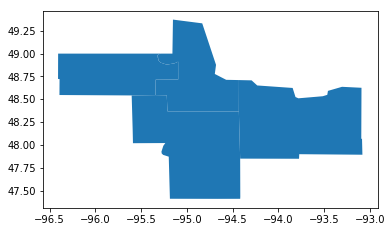That said, all of those polygons actually touch one another:

In :
counties.geometry.touches(counties.geometry).nonzero()

Out:
(array([22, 30, 40]),)

Since bounding box intersection is necessary for polygons to touch, this provides a useful filter to reduce the number of expensive polygon touches computations required.

But, since this condition is only necessary, not sufficient, this sometimes is not the case:

In :
for i, geom in enumerate(counties.geometry):
touches, = counties.geometry.touches(geom).nonzero()
sindex_hits = list(counties.sindex.intersection(geom.bounds))
sindex_hits.remove(i)
if set(touches) != set(sindex_hits):
countyname, statename = counties.iloc[i][['NAME', 'STATE_NAME']]
print('found a spatial index miss in {} County, {}!'.format(countyname,
statename))
break

found a spatial index miss in Lincoln County, Montana!


For example, in Lincoln County, MT, we see that there are spatial index misses, where bounding box intersection exists, but polygons don't intersect.

Polygons with blue stars in their center are counties whose bounding boxes overlap with our target, Lincoln County (colored light red with a blue x in the center). Red-bounded polygons are polygons that actually touch.

In :
counties.plot(color='lightgrey', edgecolor='k', linewidth=.4)
counties.iloc[[i]].plot(color='salmon', ax=plt.gca())
counties.iloc[[i]].centroid.plot(color='blue', ax=plt.gca(),
marker='x', label='focal')
counties.iloc[sindex_hits].centroid.plot(color='blue', ax=plt.gca(),
marker='*', label='sindex hits')
counties.iloc[touches].boundary.plot(color='red', ax=plt.gca(),
label='touches')
w,e,s,n = counties.iloc[sindex_hits].unary_union.buffer(.1).bounds
plt.axis((w,s,e,n))
plt.legend(loc = 'lower right', framealpha=1)
plt.xlabel("Longitude")
plt.ylabel("Latitude")
plt.show()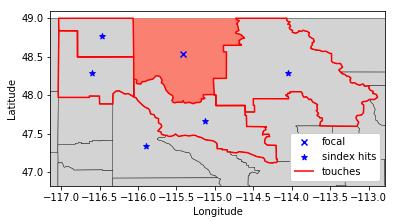On average, how efficient our spatial index is depends on the regularity of the geometries in the dataset. Highly irregular non-convex geometries will result in significantly more index misses than those where polygons are regularly spaced and evenly-sized. For US counties, we have a pretty acceptable number of tree hits:

In :
all_sindex_hits = 0
all_touches = 0
for i, geom in enumerate(counties.geometry):
touches, = counties.geometry.touches(geom).nonzero()
sindex_hits = list(counties.sindex.intersection(geom.bounds))
sindex_hits.remove(i)
all_sindex_hits += len(sindex_hits)
all_touches += len(touches)
print("{:.2f}% of spatial index intersections are also touching polygons"
"".format(all_touches/all_sindex_hits * 100))

92.54% of spatial index intersections are also touching polygons


Further, our spatial index is very good at reducing the number of polygons we use for expensive intersection operations. Instead of requiring $N^2$ touches computations (or, at best, $N^2/2$ since touches is symmetrical), we require less than one percent of the number of touches evaluations if we use the spatial index:

In :
n_hits = [len(list(counties.sindex.intersection(geom.bounds)))-1
for geom in counties.geometry]
plt.hist(n_hits, normed=True)
plt.vlines(np.mean(n_hits), *plt.gca().get_ylim(), color='cyan')
plt.text(x=9, y=.15, s='# Touches: {} \n ({:.2f}% of Naive)'.format(np.sum(n_hits),
np.sum(n_hits)/len(counties)**2*100),
fontsize=14)
plt.title("Number of Hits in Spatial Index")
plt.show()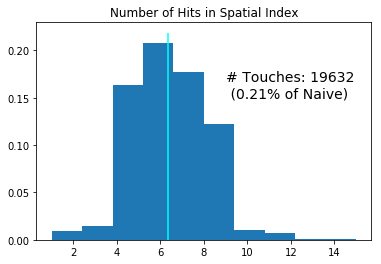So, how well does this speedup perform?

In :
def graph_sindex(gdf):
neighbors = {}
for i, geometry in enumerate(gdf.geometry):
observation_ix = gdf.iloc[i].name
hits_sindex = list(gdf.sindex.intersection(geometry.bounds))
hits = gdf.geometry.iloc[hits_sindex].touches(geometry.boundary)
these_neighbors = set(hits.index[hits].tolist())
these_neighbors.remove(observation_ix)
neighbors[observation_ix] = these_neighbors
return neighbors

In :
%%timeit -n 1
graph_sindex(counties)

6.96 s ± 273 ms per loop (mean ± std. dev. of 7 runs, 1 loop each)


From 1 minute to 6 seconds; that's an excellent improvement! Let's check if they're equal graphs, though:

In :
g1 = graph_sindex(counties)

In :
g0 == g1

Out:
True

Yep! They are!

### The Cost of GEOS point-set operations¶

While 6 seconds for a graph from ~3k polygons seems relatively long, I believe it's about as fast as you can get with using pure Python on top of shapely objects, without going directly into the GEOS object shapely wraps. Part of this is indirection of moving back and forth between the Python and the C (where GEOS is), but part of the concern is also that the topological touches relationship can be made slightly more simple for most data.

Formally, two observations touch when they share a boundary point (and no interior points). If the geometries are planar (i.e. they exist in one plane with no overlap), the only time two polygons can share a boundary point is when they share a vertex on their boundary. So, it's sufficient to examine the vertexes on the polygon boundary; when at least one point is shared, the polygons touch.

Exploiting this planarity realization, pysal, the python spatial analysis library, has special fast constructors for these kinds of graphs. They extract the vertices on the boundary of each polygon and checks when polygons share a common boundary vertex.

In :
def graph_pysal(gdf):
w = weights.Contiguity.Queen.from_dataframe(gdf)
return w.neighbors

In :
%%timeit -n 1
graph_pysal(counties)

500 ms ± 23.1 ms per loop (mean ± std. dev. of 7 runs, 1 loop each)


Whereas our first speedup dropped us quite far (from a minute to 6 seconds for a graph of the counties that tough), this speedup reduces us further still, down to under a second for the adjacency graph of 3k polygons.

Checking if they are equal, we need to ensure that neighbors in the neighbor dictionary are cast to sets:

In :
g2 = graph_pysal(counties)

In :
g2 = {k:set(v) for k,v in g2.items()}


And with this, we can check equality:

In :
g2 == g1

Out:
True

And, we can see what the adjacency graph looks like, too, using PySAL.

In :
west_is_best = counties.query("STATE_NAME in ('California', 'Oregon', 'Washington')").reset_index()
W = weights.Contiguity.Queen.from_dataframe(west_is_best)
ax = west_is_best.plot(color='lightgrey', edgecolor='k', )
f,ax = W.plot(west_is_best, ax=ax,
edge_kws=dict(color='r', linewidth=.5),
node_kws=dict(marker=''))
ax.set_axis_off()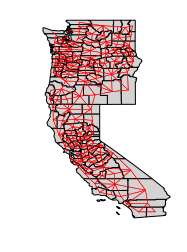# We're undergoing some renovation¶

but, if you'd like to preview this new version of pysal, install the release candidate using:

pip install --pre pysal